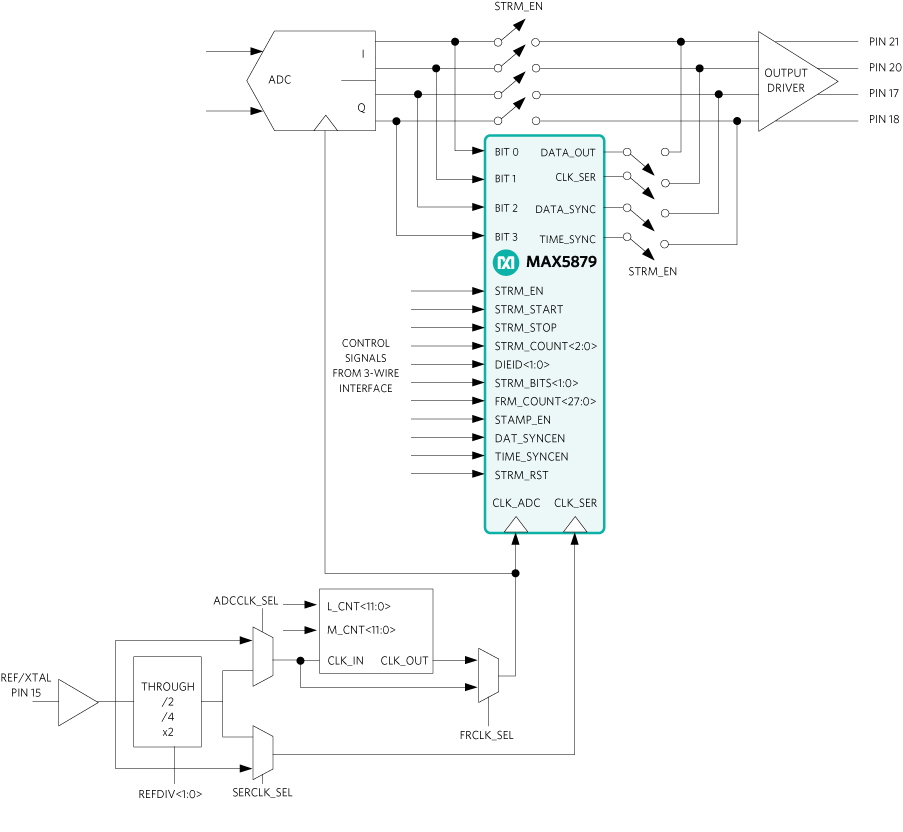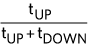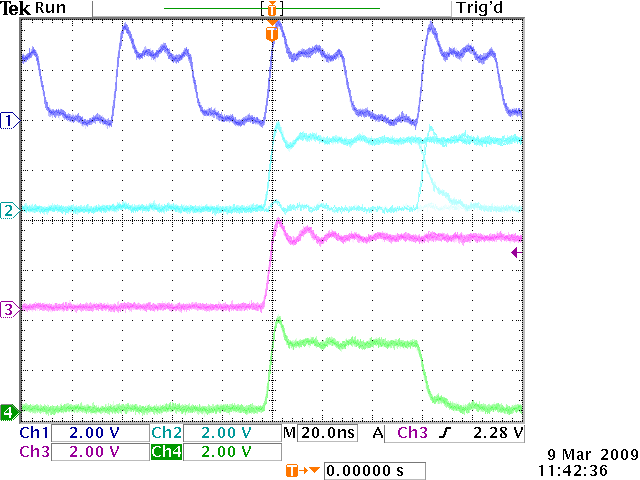# How to Configure the MAX2769C ADC Registers for DSP Interface Mode

### 要約

The MAX2769C ADC outputs support two types of baseband interface: default mode and DSP interface mode. This application note discusses how to configure the ADC registers for DSP interface mode. Refer to the MAX2769C data sheet for the default mode configuration.

### Introduction

The MAX2769C is a global navigation satellite system (GNSS) receiver covering GPS, GLONASS, Galileo, and BeiDou navigation satellite systems on a single chip. This single-conversion GNSS receiver is designed to provide high performance for industrial applications and a wide range of consumer applications, including mobile handsets.

The MAX2769C includes an on-chip ADC used to digitize the downconverted GNSS signal. The ADC outputs CMOS logic levels with one or two quantized bits for both I and Q channels or up to three quantized bits for the I channel. I and Q analog outputs are also available that bypass the on-chip ADC.

When the ADC is in use, the MAX2769C supports two types of baseband interface:

• In the default mode, I and Q samples are output on the I0 and I1 outputs and Q0 and Q1 outputs, respectively. The user can select how many bits per I and Q sample are used by register configuration. One sample is output on each of the I and Q outputs per CLK_OUT period.
• In the DSP interface mode, as many as 4 bits can be multiplexed onto a single output.

The MAX2769C data sheet explains in detail the default mode only. This application note explains how to configure the required registers for DSP interface mode.

### DSP Interface Mode

#### Overview

The purpose of the DSP interface is twofold. First, the DSP interface assembles ADC data into frames that have timing information inserted at the beginning of each frame via a frame number. Second, the DSP interface provides a means for the data serialization in the case of multibit ADC data output.

The frame counter's value is inserted into the data stream at the beginning of each frame as a timestamp. The 2 bits of the DIEID code and 2 bits of the STRM_BITS word are prefixed to a 28-bit long frame number such that the total length of the frame (time) stamp is 32 bits that are located at the beginning of each frame.

Following the frame stamp bits, GPS data is serialized into 16-bit segments of bit 0 followed by bit 1, bit 2, and bit 3. The number of bits to be serialized is controlled by the STRM_BITS word, which selects among 1-, 2-, and 4-bit cases.

#### Description

The on-chip multibit ADC digitizes the downconverted GPS signal and outputs data as four logic signals (bit 0, bit 1, bit 2, bit 3) that represent sign/magnitude, unsigned binary, or two’s complement binary data in the I and/or Q channels. Refer to the IC data sheet for a detailed description of the data format.

The resolution of the ADC can be set up to 3 bits per channel. For example, the 2-bit I and Q data in sign/magnitude format is mapped as follows: bit 0 = Sign_I, bit 1 = Mag_I, bit 2 = Sign_Q, bit 3 = Mag_Q.

The data can be serialized into 16-bit segments of bit 0 followed by bit 1, bit 2, and bit 3. The number of bits to be serialized is controlled by the STRM_BITS word, which selects among bit 0, bit 0 and bit 1, bit 0 and bit 2, bit 0, bit 1, bit 2, and bit 3 cases.

If only bit 0 is serialized, the data stream consists of bit 0 data only. If a serialization of bit 0 and bit 1 (or bit 2) is selected, the stream data pattern consists of 16 bits of bit 0 data followed by 16 bits of bit 1 (or bit 2) data, which, in turn, is followed by 16 bits of bit 0 data and so on. In this case, the serial clock must be at least twice as fast as the ADC clock.

If a 4-bit serialization of bit 0, bit 1, bit 2, bit 3 is chosen, the serial clock must be at least 4x faster than the ADC clock.

If the fractional clock divider is used, the instantaneous division ratio between serial (CLK_SER) and ADC (CLK_ADC) clocks is continuously varied under the control of the fractional clock divider. For example, if the fractional clock period equals 4.5 times the serial clock period, an average division ratio of 4.5 is achieved via a series of alternating /4 and /5 periods.

The ADC data is loaded in parallel into four holding registers that correspond to four ADC outputs. Holding registers are 16 bits long and are clocked by the ADC clock, which is a clock taken from the output of the fractional divider. At the end of the 16-bit ADC cycle, the data is transferred into four shift registers and shifted serially to the output during the next 16-bit ADC cycle. Shift registers are clocked by a serial clock that must be fast enough to shift all 64 = 4 x 16 bits of data out before the next set of data is loaded from the ADC. An all-zero pattern follows the data after all 64 bits of data are streamed to the output. A DATA_SYNC signal is used to signal the beginning of each valid 16-bit data slice. In addition, there is a TIME_SYNC signal that is output every 128 to 16,384 cycles of the ADC clock.#### 3-Wire SPI Interface Control Signals

• STRM_EN: This command configures the IC such that the DSP interface is inserted in the signal path. At the same time, all internal registers are reset.
• STRM_START: The positive edge of this command enables data streaming to the output. It also enables clock and frame sync outputs.
• STRM_STOP: The positive edge of this command disables data streaming to the output. It also disables clock and frame sync outputs.
• STRM_COUNT<2:0>: This control signal selects the length of the data (time) counter; the minimum value is 128 bits (000) and the maximum value is 16,394 bits (111).
• DIEID<1:0>: This word identifies the IC’s hardware version. The MAX2769C's default version corresponds to 00.
• STRM_BITS<1:0>: This word defines the number of bits to be streamed to the output.
00 = Bit 0
01 = Bit 0, Bit 1
10 = Bit 0, Bit 2
11 = Bit 0, Bit 1, Bit 2, Bit 3
• FRM_COUNT<27:0>. This word defines the frame number at which to start streaming. This mode is active when streaming mode is enabled by a command STRM_EN, but a command STRM_START is not received. In this case, the frame counter is reset upon the assertion of STRM_EN, and it begins its count. When the frame number reaches the value defines by FRM_COUNT, the streaming begins.
• STAMP_EN: This signal enables the insertion of the frame number at the beginning of each frame. If disabled, only the ADC data is streamed to the output.
• DAT_SYNCEN: This control signal enables sync pulses at the DATA_SYNC output. The rising edge of each pulse is coincident with the beginning of the valid data stream, which always begins at Bit 0<0> data and stops after 16, 32, or 64 cycles depending of the number of bits streamed.
• TIME_SYNCEN: This signal enables the output of time sync pulses at all times when streaming is enabled via the STRM_EN command. Otherwise, the time sync pulses are available only when data streaming is active at the output, i.e., in the time intervals bound by the STRM_START and STRM_STOP commands.
• STRM_RST: This command resets all the counters irrespective of the timing within the stream cycle.### Timing Diagram

In the DSP interface mode, the baseband is the recipient of the DATA_SYNC, TIME_SYNC, and DATA_OUT outputs of the MAX2769C. The DATA_SYNC, TIME_SYNC, and DATA_OUT signals are asserted on the rising edge of CLK_SER and need to be latched into the baseband on the falling clock edge. Figure 3 and Figure 4 show the functional timing of the DSP interface signals.TIMING VALUES TIME TYP DEVIATION tDELAY 0ns ±2ns0.5 ±0.03

The numbers in Table 1 represent the typically expected timing values and the expected maximum deviation from the standard values. Figure 5 further shows the relationship among the three signals as experienced in an actual application.

The duty cycle of the CLK_SER signal is determined predominantly by the duty cycle of the reference signal applied at the MAX2769C's pin 15 (XTAL). The numbers provided are for the case where a Rakon IT3205CE series 16.368MHz TCXO is used as the reference.

This data represents Maxim’s best effort to date to provide specific application guidance but does not express any guarantee.### Register Configurations

 BIT NAME LOCATION (0 = LSB) DEFAULT FUNCTION STRM_EN 11 0 DSP Interface for Serial Streaming of Data Enable. This bit configures the IC such that the DSP interface is inserted in the signal path. Set 1 to enable the interface or 0 to disable the interface. STRM_START 10 0 The positive edge of this command enables data streaming to the output. It also enables clock, data sync, and frame sync outputs. STRM_STOP 9 0 The positive edge of this command disables data streaming to the output. It also disables clock, data sync, and frame sync outputs. STRM_COUNT 8:6 111 Sets the length of the time counter from 128 (000) to 16384 (111). Note: The IC data sheet shows these bits as reserved, but these bits can still be controlled. STRM_BITS 5:4 01 Number of bits streamed. D[5:4] = 00: I MSB; 01: I MSB, I LSB; 10: I MSB, Q MSB; 11: I MSB, I LSB, Q MSB, Q LSB. STAMP_EN 3 1 The signal enables the insertion of the frame number at the beginning of each frame. If disabled, only the ADC data is streamed to the output. TIME_SYNCEN 2 1 This signal enables the output of the time sync pulses at all times when streaming is enabled by the STRM_EN command. Otherwise, the time sync pulses are available only when data streaming is active at the output, for example, in the time intervals bound by the STRM_START and STRM_STOP commands. DAT_SYNCEN 1 0 This control signal enables the sync pulses at the DATA_SYNC output. Each pulse is coincident with the beginning of the 16-bit data word that corresponds to a given output bit. STRM_RST 0 0 This command resets all the counters irrespective of the timing within the stream cycle.
 BIT NAME LOCATION (0 = LSB) DEFAULT FUNCTION L_CNT 27:16 256d Sets the value for the L counter. 000100000000 = 256 fractional clock divider, 100000000000 = 2048 fractional clock divider M_CNT 15:4 1563d Sets the value for the M counter. 011000011011 = 1563 fractional clock divider, 100000000 = 2048 fractional clock divider FCLKIN 3 0 Fractional clock divider. Set 1 to select the ADC clock to come from the fractional clock divider, or 0 to bypass the ADC clock from the fractional clock divider. ADCCLK 2 0 ADC clock selection. Set 0 to select the ADC and fractional divider clocks to come from the reference divider/multiplier. SERCLK 1 1 0 selects the serializer clock to come from the reference divider. MODE 0 0 DSP interface mode selection, set to 1 for standard mode operation.